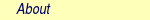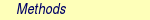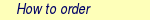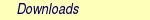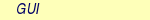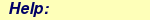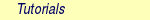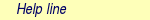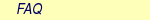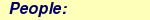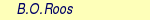MOLCAS manual:Next: 8.49 The Basis Set Up: 8. Programs Previous: 8.47 surfacehop

Subsections

# 8.48 vibrot

The program VIBROT is used to compute a vibration-rotation spectrum for a diatomic molecule, using as input a potential computed over a grid. The grid should be dense around equilibrium (recommended spacing 0.05 au) and should extend to large distance (say 50 au) if dissociation energies are computed.

The potential is fitted to an analytical form using cubic splines. The ro-vibrational Schrödinger equation is then solved numerically (using Numerov's method) for one vibrational state at a time and for a number of rotational quantum numbers as specified by input. The corresponding wave functions are stored on file VIBWVS for later use. The ro-vibrational energies are analyzed in terms of spectroscopic constants. Weakly bound potentials can be scaled for better numerical precision.

The program can also be fed with property functions, such as a dipole moment curve. Matrix elements over the ro-vib wave functions for the property in question are then computed. These results can be used to compute IR intensities and vibrational averages of different properties.

VIBROT can also be used to compute transition properties between different electronic states. The program is then run twice to produce two files of wave functions. These files are used as input in a third run, which will then compute transition matrices for input properties. The main use is to compute transition moments, oscillator strengths, and lifetimes for ro-vib levels of electronically excited states. The asymptotic energy difference between the two electronic states must be provided using the ASYMptotic keyword.

## 8.48.1 Dependencies

The VIBROT is free-standing and does not depend on any other program.

## 8.48.2 Files

### 8.48.2.1 Input files

The calculation of vibrational wave functions and spectroscopic constants uses no input files (except for the standard input). The calculation of transition properties uses VIBWVS files from two preceding VIBROT runs, redefined as VIBWVS1 and VIBWVS2.

### 8.48.2.2 Output files

VIBROT generates the file VIBWVS with vibrational wave functions for each v and J quantum number, when run in the wave function mode. If requested VIBROT can also produce files VIBPLT with the fitted potential and property functions for later plotting.

## 8.48.3 Input

This section describes the input to the VIBROT program in the MOLCAS program system. The program name is

&VIBROT

### 8.48.3.1 Keywords

The first keyword to VIBROT is an indicator for the type of calculation that is to be performed. Two possibilities exist:
 Keyword Meaning ROVIbrational spectrum VIBROT will perform a vib-rot analysis and compute spectroscopic constants. TRANsition moments VIBROT will compute transition moment integrals using results from two previous calculations of the vib-rot wave functions. In this case the keyword Observable should be included, and it will be interpreted as the transition dipole moment.
Note that only one of the above keywords can be used in a single calculation. If none is given the program will only process the input section.

After this first keyword follows a set of keywords, which are used to specify the run. Most of them are optional.

The compulsory keywords are:
 Keyword Meaning ATOMs Gives the mass of the two atoms. Write mass number (an integer) and the chemical symbol Xx, in this order, for each of the two atoms in free format. If the mass numbers is zero for any atom, the mass of the most abundant isotope will be used. All isotope masses are stored in the program. You may introduce your own masses by giving a negative integer value to the mass number (one of them or both). The masses (in unified atomic mass units, or Da) are then read on the next (or next two) entry(ies). The isotopes of hydrogen can be given as H, D, or T. POTEntial Gives the potential as an arbitrary number of lines. Each line contains a bond distance (in au) and an energy value (in au). A plot file of the potential is generated if the keyword Plot is added after the last energy input. One more entry should then follow with three numbers specifying the start and end value for the internuclear distance and the distance between adjacent plot points. This input must only be given together with the keyword RoVibrational spectrum.

In addition you may want to specify some of the following optional input:
 Keyword Meaning TITLe One single title line GRID The next entries give the number of grid points used in the numerical solution of the radial Schrödinger equation. The default value is 199. The maximum value that can be used is 4999. RANGe The next entry contains two distances Rmin and Rmax (in au) specifying the range in which the vibrational wave functions will be computed. The default values are 1.0 and 5.0 au. Note that these values most often have to be given as input since they vary considerably from one case to another. If the range specified is too small, the program will give a message informing the user that the vibrational wave function is large outside the integration range. VIBRational The next entry specifies the number of vibrational quanta for which the wave functions and energies are computed. Default value is 3. ROTAtional The next entry specifies the range of rotational quantum numbers. Default values are 0 to 5. If the orbital angular momentum quantum number () is non zero, the lower value will be adjusted toif the start value given in input is smaller than. ORBItal The next entry specifies the value of the orbital angular momentum (0,1,2, etc). Default value is zero. SCALe This keyword is used to scale the potential, such that the binding energy is 0.1 au. This leads to better precision in the numerical procedure and is strongly advised for weakly bound potentials. NOSPectroscopic Only the wave function analysis will be carried out but not the calculation of spectroscopic constants. OBSErvable This keyword indicates the start of input for radial functions of observables other than the energy, for example the dipole moment function. The next line gives a title for this observable. An arbitrary number of input lines follows. Each line contains a distance and the corresponding value for the observable. As for the potential, this input can also end with the keyword Plot, to indicate that a file of the function for later plotting is to be constructed. The next line then contains the minimum and maximum R-values and the distance between adjacent points. When this input is given with the top keyword RoVibrational spectrum the program will compute matrix elements for vibrational wave functions of the current electronic state. Transition moment integrals are instead obtained when the top keyword is Transition moments. In the latter case the calculation becomes rather meaningless if this input is not provided. The program will then only compute the overlap integrals between the vibrational wave functions of the two states. The keyword Observable can be repeated up to ten times in a single run. All observables should be given in atomic units. TEMPerature The next entry gives the temperature (in K) at which the vibrational averaging of observables will be computed. The default is 300 K. STEP The next entry gives the starting value for the energy step used in the bracketing of the eigenvalues. The default value is 0.004 au (88 cm-1). This value must be smaller than the zero-point vibrational energy of the molecule. ASYMptotic The next entries specifies the asymptotic energy difference between two potential curves in a calculation of transition matrix elements. The default value is zero atomic units. ALLRotational By default, when the Transition moments keyword is given, only the transitions between the lowest rotational level in each vibrational state are computed. The keyword AllRotational specifies that the transitions between all the rotational levels are to be included. Note that this may result in a very large output file. PRWF Requests the vibrational wave functions to be printed in the output file.

### 8.48.3.2 Input example

&VIBROT
RoVibrational  spectrum
Title  =  Vib-Rot  spectrum  for  FeNi
Atoms  =  0  Fe  0  Ni
Potential
1.0  -0.516768
1.1  -0.554562
[...]
Plot  =  1.0  10.0  0.1
Grid  =  150
Range  =  1.0  10.0
Vibrations  =  10
Rotations  =  2  10
Orbital  =  2
Observable
Dipole  Moment
1.0  0.102354
1.1  0.112898
[...]
Plot  =  1.0  10.0  0.1

Comments: The vibrational-rotation spectrum for FeNi will be computed using the potential curve given in input. The 10 lowest vibrational levels will be obtained and for each level the rotational states in the range J=2 to 10. The vib-rot matrix elements of the dipole function will also be computed. A plot file of the potential and the dipole function will be generated. The masses for the most abundant isotopes of Fe and Ni will be selected.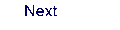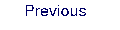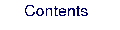Next: 8.49 The Basis Set Up: 8. Programs Previous: 8.47 surfacehop